# MATH1312 Regression Analysis Proof Reading Service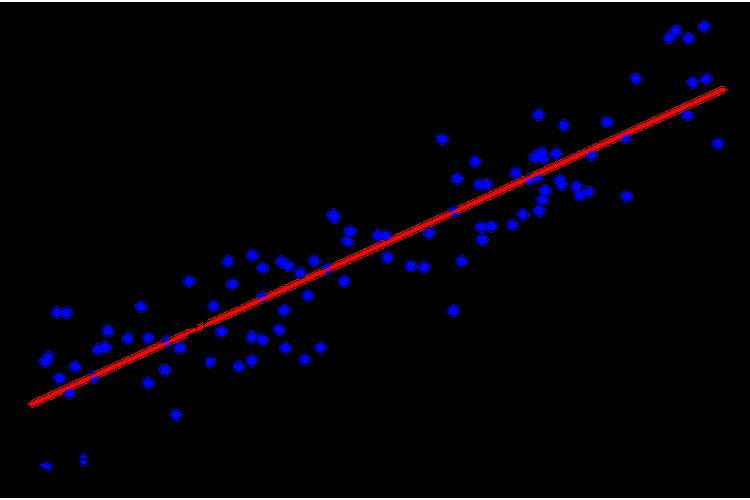## 1 A.

### Scatter plot: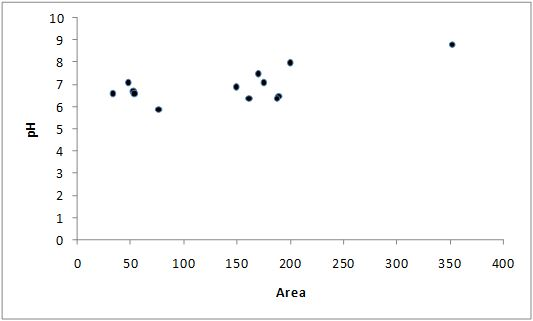From the above plot, it can be identified that:

The pH value of around 6.5 is the most prevalent in the different lakes irrespective of their sizes.

For smaller lakes, the pH value is lying to acidic side (i.e., having a pH value less than 7), while the lakes that are bigger are both acidic as well as basic.

The largest lake is the one at the highest pH value having an exceptionally high value for a sigficantly larger area as compared to the other lakes.

### 1 B.

For using the principal of least squares for fitting a regression line to the data where pH is the dependent variable (y) and area is the independent variable (x), following calculation is needed to be done:

 Observation Area (x) ph (y) xy x2 y2 1 33 6.6 217.8 1089 43.56 2 161 6.4 1030.4 25921 40.96 3 189 6.5 1228.5 35721 42.25 4 149 6.9 1028.1 22201 47.61 5 47 7.1 333.7 2209 50.41 6 170 7.5 1275 28900 56.25 7 352 8.8 3097.6 123904 77.44 8 187 6.4 1196.8 34969 40.96 9 76 5.9 448.4 5776 34.81 10 52 6.7 348.4 2704 44.89 11 175 7.1 1242.5 30625 50.41 12 53 6.6 349.8 2809 43.56 13 200 8.0 1600 40000 64 Sum 1844 90.5 13397 356828 637.11

From the above table, Σx = 1844, Σy = 90.5, Σxy = 13397, Σx2 = 356828, Σy2 = 637.11 n is the sample size (13, in our case).

We will use the following formula for finding a and b, where the regression line is y = a + bx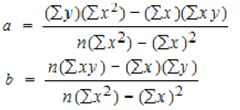a= 6.13, b= 0.006

Hence the regression line is y = 6.127 + 0.005x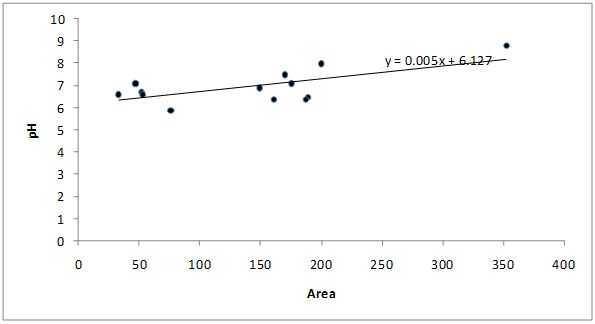### 1C.

For the ANOVA test, following table is needed to be calculated:

 DF SS MS F Regression 1 SSR= ∑ (yi−y)2 MSR=SSR/1 F∗=MSR/MSE Residual n-2 SSE=∑(yi−yi)2 MSE=SSE/(n−2) Total n-1 SSTO=∑(yi−y)2

Whereyiis the estimated valued through the regression line

Following is the calculation for the table

 Observation Area (x) ph (y) xy x^2 y^2 estimated y (y-estimated y)^2 (estimated y- avg. of y)^2 (y-avg. of y)^2 1 33 6.6 217.8 1089 43.56 6.32 0.08 0.41 0.13 2 161 6.4 1030.4 25921 40.96 7.07 0.46 0.01 0.32 3 189 6.5 1228.5 35721 42.25 7.24 0.55 0.08 0.21 4 149 6.9 1028.1 22201 47.61 7.00 0.01 0.00 0.00 5 47 7.1 333.7 2209 50.41 6.40 0.48 0.31 0.02 6 170 7.5 1275 28900 56.25 7.13 0.14 0.03 0.29 7 352 8.8 3097.6 123904 77.44 8.20 0.36 1.53 3.38 8 187 6.4 1196.8 34969 40.96 7.23 0.68 0.07 0.32 9 76 5.9 448.4 5776 34.81 6.57 0.46 0.15 1.13 10 52 6.7 348.4 2704 44.89 6.43 0.07 0.28 0.07 11 175 7.1 1242.5 30625 50.41 7.16 0.00 0.04 0.02 12 53 6.6 349.8 2809 43.56 6.44 0.03 0.27 0.13 13 200 8.0 1600 40000 64 7.30 0.48 0.12 1.08 Sum 1844 90.5 13397 356828 637.11 90.51 3.80 3.29 7.09

Following is the ANOVA table:

 DF SS MS F Regression 1 3.291011 3.291011 9.527217 Residual 11 3.799758 0.345433 Total 12 7.090769

We are testing the null hypothesis H0: b = 0 against the alternative hypothesis Ha: b ≠ 0.

If b = 0 then F = 1

If F > 1 then b ≠ 0, which means there is a linear relationship

In view of the fact there F = 9.52, we can say there is a linear relationship

### 1D.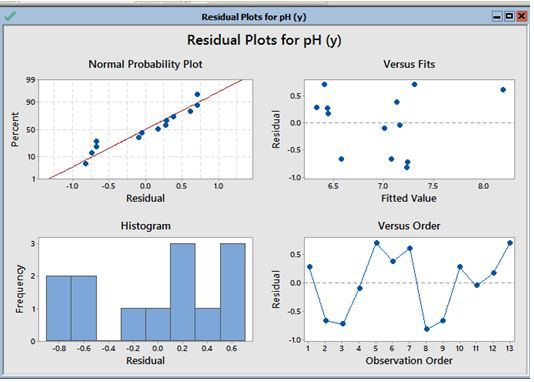Following are the assumptions of Regression

linearity and additivity – This can be seen from normal probability plot

statistical independence – This can be seen from the Versus fits

homoscedasticity - This can be seen from the Versus Orders

normality - This can be seen from the histogram

### 1E.

The regression line is y = 6.128 + 0.00588x

If x = 2050 then pH = 18.18

For developing a 99% CI for this prediction, following is the computation needed: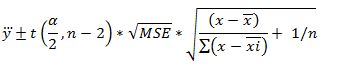Computing the values, following is the confidence interval (6.88, 29.47)

### 2 A.

For using the principal of least squares for fitting a regression line to the data where pressure is the dependent variable (y) and steam is the independent variable (x), following calculation is needed to be done:

 Observation steam (x) pressure (y) xy x^2 y^2 1 35.3 10.98 387.59 1,246.09 120.56 2 29.7 11.13 330.56 882.09 123.88 3 30.8 12.51 385.31 948.64 156.50 4 58.8 8.4 493.92 3,457.44 70.56 5 61.4 9.27 569.18 3,769.96 85.93 6 71.3 8.73 622.45 5,083.69 76.21 7 74.4 6.36 473.18 5,535.36 40.45 8 76.7 8.5 651.95 5,882.89 72.25 9 70.7 7.82 552.87 4,998.49 61.15 10 57.5 9.14 525.55 3,306.25 83.54 11 46.4 8.24 382.34 2,152.96 67.90 12 28.9 12.19 352.29 835.21 148.60 13 28.1 11.88 333.83 789.61 141.13 14 39.1 9.57 374.19 1,528.81 91.58 15 46.8 10.94 511.99 2,190.24 119.68 16 48.5 9.58 464.63 2,352.25 91.78 17 59.3 10.09 598.34 3,516.49 101.81 18 70 8.11 567.70 4,900.00 65.77 19 70 6.83 478.10 4,900.00 46.65 20 74.5 8.88 661.56 5,550.25 78.85 21 72.1 7.68 553.73 5,198.41 58.98 22 58.1 8.47 492.11 3,375.61 71.74 23 44.6 8.86 395.16 1,989.16 78.50 24 33.4 10.36 346.02 1,115.56 107.33 25 28.6 11.08 316.89 817.96 122.77 Sum 1315 235.6 11821.43 76323.42 2284.11

From the above table, Σx = 1315, Σy = 235.6, Σxy = 11821.43, Σx2 = 76323.42, Σy2 = 2284.11 n is the sample size (25, in our case).

We will use the following formula for finding a and b, where the regression line is y = a + bx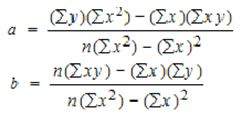a= 13.62, b= -0.079

Hence the regression line is y = 13.62 - 0.079x

The least square estimates for constant is 13.62 and slope is  -0.079

### 2B.

Following are the residuals

 Observation steam (x) pressure (y) xy x^2 y^2 estimated y Residual 1 35.3 10.98 387.59 1,246.09 120.56 10.81 0.17 2 29.7 11.13 330.56 882.09 123.88 11.25 -0.12 3 30.8 12.51 385.31 948.64 156.50 11.16 1.35 4 58.8 8.4 493.92 3,457.44 70.56 8.93 -0.53 5 61.4 9.27 569.18 3,769.96 85.93 8.72 0.55 6 71.3 8.73 622.45 5,083.69 76.21 7.93 0.80 7 74.4 6.36 473.18 5,535.36 40.45 7.68 -1.32 8 76.7 8.5 651.95 5,882.89 72.25 7.50 1.00 9 70.7 7.82 552.87 4,998.49 61.15 7.98 -0.16 10 57.5 9.14 525.55 3,306.25 83.54 9.03 0.11 11 46.4 8.24 382.34 2,152.96 67.90 9.92 -1.68 12 28.9 12.19 352.29 835.21 148.60 11.32 0.87 13 28.1 11.88 333.83 789.61 141.13 11.38 0.50 14 39.1 9.57 374.19 1,528.81 91.58 10.50 -0.93 15 46.8 10.94 511.99 2,190.24 119.68 9.89 1.05 16 48.5 9.58 464.63 2,352.25 91.78 9.75 -0.17 17 59.3 10.09 598.34 3,516.49 101.81 8.89 1.20 18 70 8.11 567.70 4,900.00 65.77 8.03 0.08 19 70 6.83 478.10 4,900.00 46.65 8.03 -1.20 20 74.5 8.88 661.56 5,550.25 78.85 7.68 1.20 21 72.1 7.68 553.73 5,198.41 58.98 7.87 -0.19 22 58.1 8.47 492.11 3,375.61 71.74 8.98 -0.51 23 44.6 8.86 395.16 1,989.16 78.50 10.06 -1.20 24 33.4 10.36 346.02 1,115.56 107.33 10.96 -0.60 25 28.6 11.08 316.89 817.96 122.77 11.34 -0.26 Sum 1315 235.6 11821.432 76323.42 2284.1102 235.6 -1.6E-14

### 2C.

For the ANOVA table following are needed to be calculated:

 Observation steam (x) pressure (y) xy x^2 y^2 estimated y (y-estiamted y)^2 (estimated y- avg. of y)^2 (y-avg. of y)^2 Residual 1 35.3 10.98 387.59 1,246.09 120.56 10.81 0.03 1.91 2.42 0.17 2 29.7 11.13 330.56 882.09 123.88 11.25 0.02 3.35 2.91 -0.12 3 30.8 12.51 385.31 948.64 156.50 11.17 1.81 3.03 9.52 1.34 4 58.8 8.4 493.92 3,457.44 70.56 8.93 0.28 0.24 1.05 -0.53 5 61.4 9.27 569.18 3,769.96 85.93 8.72 0.30 0.49 0.02 0.55 6 71.3 8.73 622.45 5,083.69 76.21 7.93 0.63 2.22 0.48 0.80 7 74.4 6.36 473.18 5,535.36 40.45 7.69 1.76 3.02 9.39 -1.33 8 76.7 8.5 651.95 5,882.89 72.25 7.50 1.00 3.69 0.85 1.00 9 70.7 7.82 552.87 4,998.49 61.15 7.98 0.03 2.08 2.57 -0.16 10 57.5 9.14 525.55 3,306.25 83.54 9.03 0.01 0.15 0.08 0.11 11 46.4 8.24 382.34 2,152.96 67.90 9.92 2.82 0.25 1.40 -1.68 12 28.9 12.19 352.29 835.21 148.60 11.32 0.76 3.58 7.65 0.87 13 28.1 11.88 333.83 789.61 141.13 11.38 0.25 3.83 6.03 0.50 14 39.1 9.57 374.19 1,528.81 91.58 10.50 0.87 1.16 0.02 -0.93 15 46.8 10.94 511.99 2,190.24 119.68 9.89 1.11 0.22 2.30 1.05 16 48.5 9.58 464.63 2,352.25 91.78 9.75 0.03 0.11 0.02 -0.17 17 59.3 10.09 598.34 3,516.49 101.81 8.89 1.44 0.28 0.44 1.20 18 70 8.11 567.70 4,900.00 65.77 8.04 0.01 1.92 1.73 0.07 19 70 6.83 478.10 4,900.00 46.65 8.04 1.46 1.92 6.73 -1.21 20 74.5 8.88 661.56 5,550.25 78.85 7.68 1.45 3.05 0.30 1.20 21 72.1 7.68 553.73 5,198.41 58.98 7.87 0.04 2.42 3.04 -0.19 22 58.1 8.47 492.11 3,375.61 71.74 8.99 0.27 0.19 0.91 -0.52 23 44.6 8.86 395.16 1,989.16 78.50 10.06 1.45 0.41 0.32 -1.20 24 33.4 10.36 346.02 1,115.56 107.33 10.96 0.36 2.35 0.88 -0.60 25 28.6 11.08 316.89 817.96 122.77 11.34 0.07 3.67 2.74 -0.26 Sum 1315 235.6 11821.432 76323.42 2284.1102 235.638 18.2234617 45.5596905 63.8158 -0.038
 DF SS MS F Regression 1 SSR= ∑ (yi−y)2 MSR=SSR/1 F∗=MSR/MSE Residual n-2 SSE=∑(yi−yi)2 MSE=SSE/(n−2) Total n-1 SSTO=∑(yi−y)2

Whereyiis the estimated valued through the regression line

 df SS MS F Regression 1 45.5924 45.5924 57.54279 Residual 23 18.2234 0.792322 Total 24 63.8158

The ANOVA table us used for test hypotheses about the regression linearity or population means. When the null hypothesis of equal means is true, the two mean squares estimate the same quantity (error variance), and should be of approximately equal magnitude. In other words, their ratio should be close to 1.

### 2D.

Following is the formula for computing correlation coefficient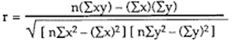Replacing the value, r= -0.845

Coefficient of determination is r2  = 0.714

### 2E.

STD for

 Predictor Error Slope Constant Constant -62.95 10.175 -6.142 Waist 0.874 0.108 8.123 STD 4.549 8.86 7.86

### 2F.

For slope

We are testing the null hypothesis H0: b = 0 against the alternative hypothesis Ha: b ≠ 0.

If b = 0 then F = 1

If F > 1 then b ≠ 0, which means there is a linear relationship

In view of the fact there F = 57.54, we can say the slope is significant

### 2G.

The confidence interval for Slope is (b1 is slope of the regression line, σb1is standard error estimate  (SE))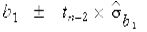Where

SE= sqrt [ Σ(yi - ?i)2 / (n - 2) ] / sqrt [ Σ(xi - x)2 ]

Computing the value the CI is (-0.109, -0.050)

### 3A.

Fo scatterplot of data following steps are followed in MInitab:

Choose Graph > Scatterplot.

Choose Simple, then click OK.

Under Y variables, select pressure as Y variable.

Under X variables, select steam as X variable.

Click OK.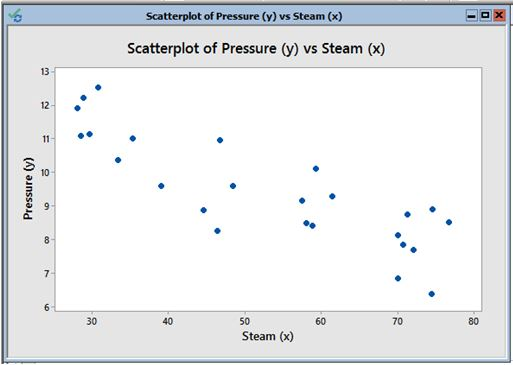There seems to be a negative correlation between the variables as can be seen from the scatterplot

### 3 B.

For linear regression, following are the steps which are taken

Choose Stat > Regression > Fitted Line Plot.

In Response (Y), enter steam.

In Predictor (X), enter pressure.

Under Type of Regression Model, choose linear.

Click OK in each dialog box.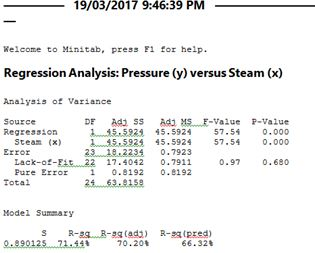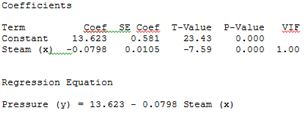For calculating the residuals, following are the steps:

Select Stat >> Regression >> Fit Regression Model ...

Specify the response and the predictor(s).

Under Graphs...

Under Residuals for Plots, select Regular

Under Residuals Plots, select Residuals versus fits.

Select OK.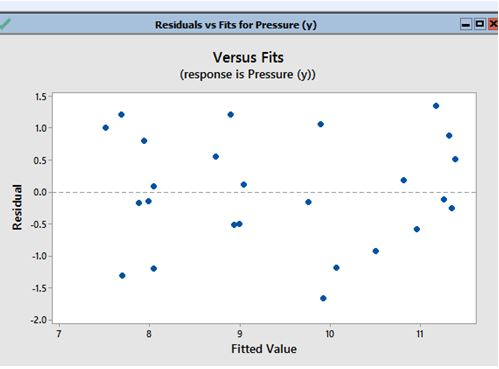For ANOVA table, Stat > ANOVA > General Linear Model > Fit General Linear Model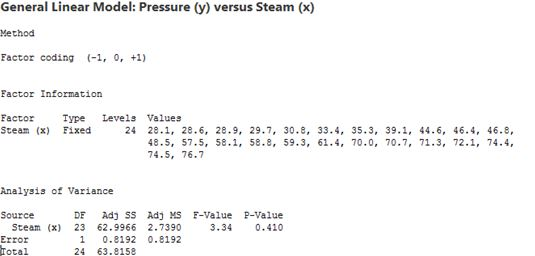For correlation coefficient, Click “Stat”, then click “Basic Statistics” and then click “Correlation.”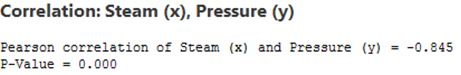Coefficient of determination is r2  = 0.714

From the output of regression: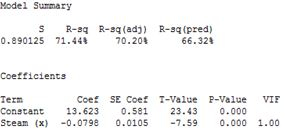As we can see the P value is 0 for both of the constant and slope. This means that null hypothesis for both can be rejected which stated the constant = 0 and slope – 0

From the output of regression the CI can be identified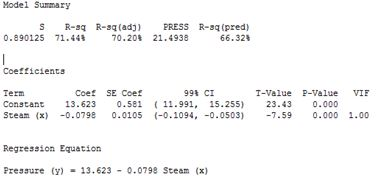### 3C.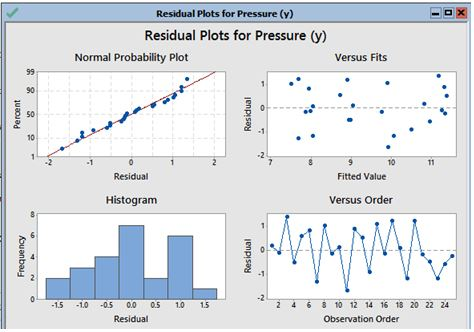linearity and additivity – This can be seen from normal probability plot

statistical independence – This can be seen from the Versus fits

homoscedasticity - This can be seen from the Versus Orders

normality - This can be seen from the histogram